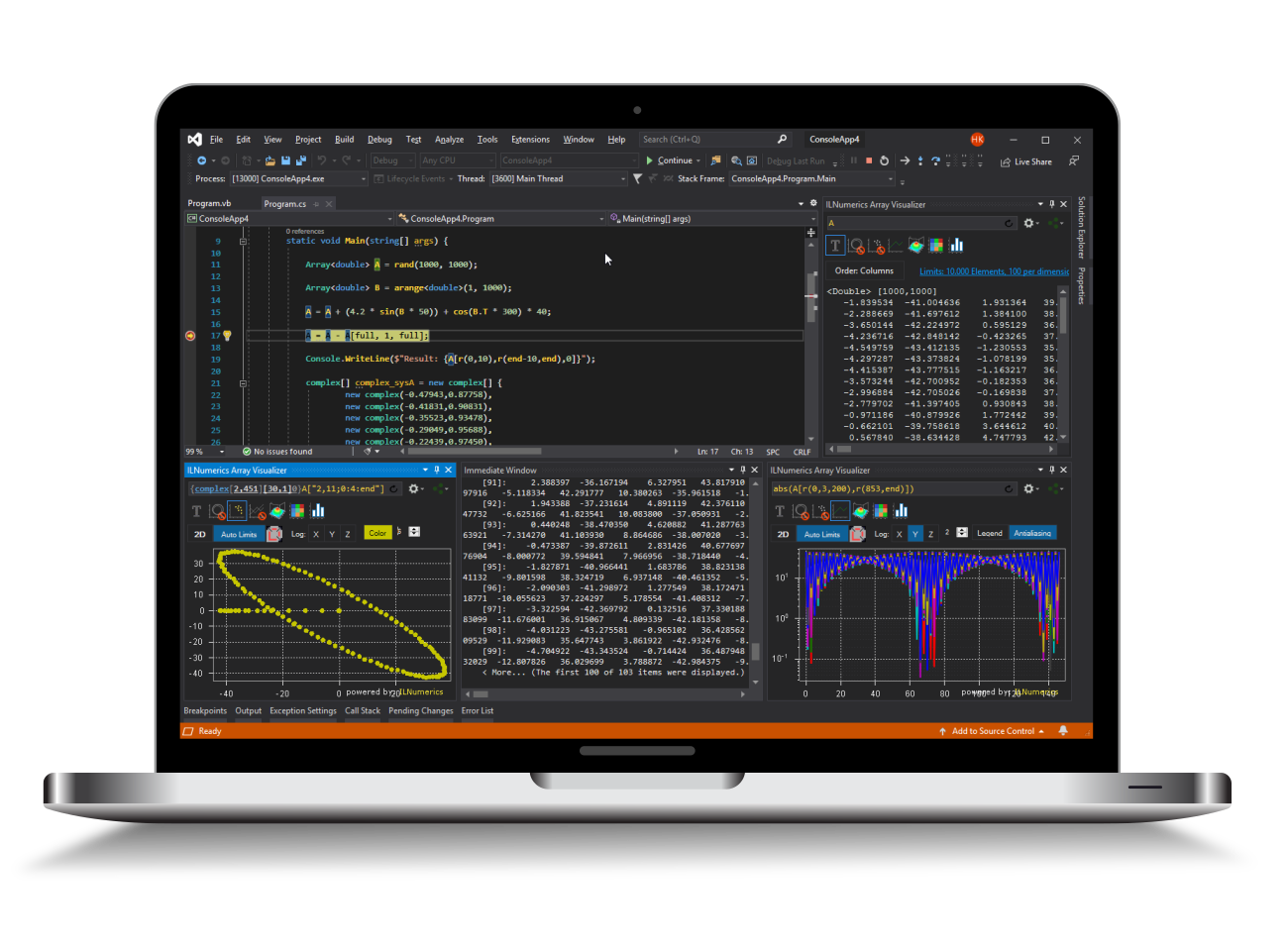Industrial Data Science
in C# and .NET:
Simple. Fast. Reliable.ILNumerics - Technical Computing

Modern High Performance Tools for Technical

Computing and Visualization in Industry and Science

tgt

# Color mapped 3D Bar Plots

BarPlotEx combines the functionalities of BarPlot and Surface. It creates 3D color mapped bar plots that visualize arbitrary rectangular grids in 3D space. Each bar is color mapped by transforming its numeric values into a colors.

Note: The property "Axis" of BarPlot does not exist in BarPlotEx. Instead, the plot cube axis are customizable using direct access. See Axis Configuration.

On this page the following BarPlotEx features are described:

## Simple Colormapped Bar Plots

The handling of BarPlotEx is as easy as the handling of Surface. Simply create a new BarPlotEx object by entering the following expression:

• public BarPlotEx(Z, X, Y, colormap, tag);

Provide the constructor with the x, y and z coordinates of your bars. The parameter Z corresponds to the height of a bar. In contrast, to the parameters X and Y, Z is mandatory. Z can either be a scalar (as a result only one bar is drawn), a vector or a matrix. If only Z is provided X and Y are calculated automatically. For more information, visit the ApiDoc.

Example : A simple color mapped bar plot.

X and Y coordinates can also be defined as scalars, vectors or matrices. Possible combinations are listed below:

• If Z is a scalar, then X and Y can only be defined as scalars.
• If Z is a row vector of length $m$, then X can be defined as a scalar and Y can be defined as a vector of length $m$.
• If Z is a column vector of length $n$, then X can be defined as a vector of length $n$, Y can be defined as a scalar.
• If Z is matrix size [$m$ x $n$], then X can be defined as a vector of length $n$, Y can also be defined as a vector of length $m$.

Example : Using X an Y to determine Z matrix positioning.

### Colormap and Border Color Settings

By default, the colormap is set to 'ILNumerics'. However, if needed, it is also possible to select a different colormap provided in Colormaps Enumeration. Assign a desired value to the parameter colormap before creating your object or modify the property Colormap at any time once your object exists. All properties changed at run time go into effect after calling the Configure() method.

Next to colormap settings, you can also get access to the border color as shown in the example below.

Example : Modifying Colormap setting.

### Color Mode Setting

Additionally, you can also set the property ColorMode. You can choose from the two values Colormapped, which is the default value or Solidmapped.

Colormapped - a default color mode state, where each bar is colored according to a given color map ranging from the minimal Z value to the maximal Z value.

Example : Colormapped color mode setting.

Solidmapped - a color mode state, where each bar is solid colored according to a given color map ranging from the minimal X value to the maximal X value.

Example : Solidmapped color mode setting.

## BarPlotEx Customization

Although BarPlotEx is an extended object of BarPlot, its common purpose is to visualize data grids similar to Surface. Besides, BarPlotEx has less parameters than BarPlot.

### Bar Width Setting

The property BarWidth can be determined inside the constructor or after creating the BarPlotEx object. The bar width determines the width of all bars and must be a scalar of the type float. It has a range of 0.0f and 1.0f. A value of 1.0f correponds to adjacent bars within a group. The default value is set to 0.8f.

Example : Bar Width Setting.

### Base Value Setting

The property BaseValue determines a baseline location, specified as a numeric scalar value. Please read the ApiDoc for more information.

Example : Base Value Setting

### Using Lighting

The property UseLigthting sets the rendering parameter to true or false. By default UseLighting is set to false. This means that the colormapped 3D bar plot will be rendered without lighting.

Example : Setting Lighting to on.

## Update and Configure Methods

The values provided to the BarPlotEx constructor can be changed at any time by using the Update() method. The Update() method accepts the same parameters as the constructor. Please be aware that the coordinates of the grid points have to be layed out as matrices in the following order Z, X and Y.

After setting all properties of BarPlotEx object, it is necessary to call the Configure() method to affect all property changes.

Example : Using the Update() and Configure() method.

## Further Reading

For more information check out: